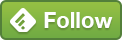Sep 15 Exploratory Data Analysis Of Google Analytics Data In R Studio

Came across this nice e-book that addresses Google Analytics data in R Studio. This blog post uses/modifies the code from: https://michalbrys.gitbooks.io/r-google-analytics/content/chapter4/exploratory_data_analysis.html

The idea of running this code is to provide the summary metrics about the underlying dataset before doing a drill-down.

In this R script, we’re using the 2018 YTD data with dimensions = date, metric = sessions.

If you plot the data, you can immediately see some variability in the daily

You can now run the min/max queries to find out the range.

Let’s say we wanted to find out dates where sessions = 0. In the subset function, we’re running the parameter, sessions = 0.

#days with 0 sessions

You can now run a count on this data by running a conditional NRow function. This only counts rows where sessions = 0.

#conditional row count only
#where sessions were 0 for that particular date

If you want to quickly know the summary stats for this data, you can run the summary function to provide this.

By running this, you now the min sessions = 0 was on 1st Jan (surprise, surprise). Max was on 13th Sep [shows that the traffic is increasing]. Mean/Median values are comparable with 7.2 and 7 while 50% of the website’s traffic values fall within 3 and 11 sessions [2nd Quartile - 3rd Quartile data]

Full code below:

ga_auth()
ga_account_list()

ga_id <- 1234567

date_range = c("2018-01-01","2018-09-15"),
metrics = c("sessions"),
dimensions = c("date"),
anti_sample = TRUE)

#code modified from
#min and max sessions received

#days with 0 sessions
#conditional row count only where sessions
#were 0 for that particular date

#count days with 0 sessions

#summary data

#when did max traffic hit the site?

#combining max number of sessions with
#the date it was received